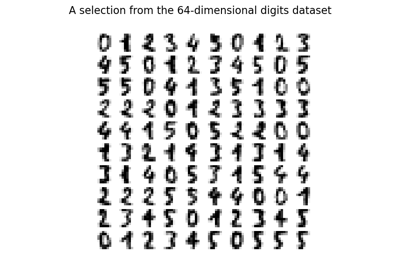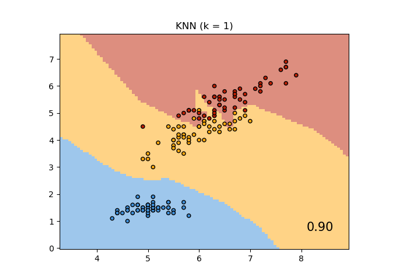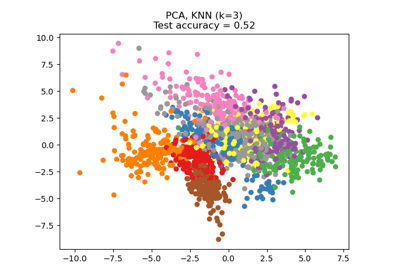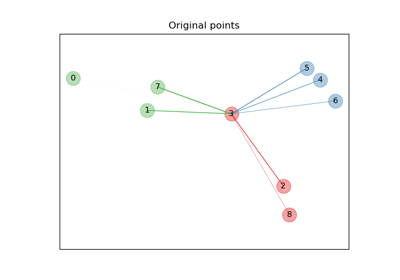# sklearn.neighbors.NeighborhoodComponentsAnalysis¶

class sklearn.neighbors.NeighborhoodComponentsAnalysis(n_components=None, *, init='auto', warm_start=False, max_iter=50, tol=1e-05, callback=None, verbose=0, random_state=None)[source]

Neighborhood Components Analysis.

Neighborhood Component Analysis (NCA) is a machine learning algorithm for metric learning. It learns a linear transformation in a supervised fashion to improve the classification accuracy of a stochastic nearest neighbors rule in the transformed space.

Read more in the User Guide.

Parameters:
n_componentsint, default=None

Preferred dimensionality of the projected space. If None it will be set to n_features.

init{‘auto’, ‘pca’, ‘lda’, ‘identity’, ‘random’} or ndarray of shape (n_features_a, n_features_b), default=’auto’

Initialization of the linear transformation. Possible options are 'auto', 'pca', 'lda', 'identity', 'random', and a numpy array of shape (n_features_a, n_features_b).

• 'auto'

Depending on n_components, the most reasonable initialization will be chosen. If n_components <= n_classes we use 'lda', as it uses labels information. If not, but n_components < min(n_features, n_samples), we use 'pca', as it projects data in meaningful directions (those of higher variance). Otherwise, we just use 'identity'.

• 'pca'

n_components principal components of the inputs passed to fit will be used to initialize the transformation. (See PCA)

• 'lda'

min(n_components, n_classes) most discriminative components of the inputs passed to fit will be used to initialize the transformation. (If n_components > n_classes, the rest of the components will be zero.) (See LinearDiscriminantAnalysis)

• 'identity'

If n_components is strictly smaller than the dimensionality of the inputs passed to fit, the identity matrix will be truncated to the first n_components rows.

• 'random'

The initial transformation will be a random array of shape (n_components, n_features). Each value is sampled from the standard normal distribution.

• numpy array

n_features_b must match the dimensionality of the inputs passed to fit and n_features_a must be less than or equal to that. If n_components is not None, n_features_a must match it.

warm_startbool, default=False

If True and fit has been called before, the solution of the previous call to fit is used as the initial linear transformation (n_components and init will be ignored).

max_iterint, default=50

Maximum number of iterations in the optimization.

tolfloat, default=1e-5

Convergence tolerance for the optimization.

callbackcallable, default=None

If not None, this function is called after every iteration of the optimizer, taking as arguments the current solution (flattened transformation matrix) and the number of iterations. This might be useful in case one wants to examine or store the transformation found after each iteration.

verboseint, default=0

If 0, no progress messages will be printed. If 1, progress messages will be printed to stdout. If > 1, progress messages will be printed and the disp parameter of scipy.optimize.minimize will be set to verbose - 2.

random_stateint or numpy.RandomState, default=None

A pseudo random number generator object or a seed for it if int. If init='random', random_state is used to initialize the random transformation. If init='pca', random_state is passed as an argument to PCA when initializing the transformation. Pass an int for reproducible results across multiple function calls. See Glossary.

Attributes:
components_ndarray of shape (n_components, n_features)

The linear transformation learned during fitting.

n_features_in_int

Number of features seen during fit.

New in version 0.24.

n_iter_int

Counts the number of iterations performed by the optimizer.

random_state_numpy.RandomState

Pseudo random number generator object used during initialization.

feature_names_in_ndarray of shape (n_features_in_,)

Names of features seen during fit. Defined only when X has feature names that are all strings.

New in version 1.0.

sklearn.discriminant_analysis.LinearDiscriminantAnalysis

Linear Discriminant Analysis.

sklearn.decomposition.PCA

Principal component analysis (PCA).

References



J. Goldberger, G. Hinton, S. Roweis, R. Salakhutdinov. “Neighbourhood Components Analysis”. Advances in Neural Information Processing Systems. 17, 513-520, 2005. http://www.cs.nyu.edu/~roweis/papers/ncanips.pdf



Wikipedia entry on Neighborhood Components Analysis https://en.wikipedia.org/wiki/Neighbourhood_components_analysis

Examples

>>> from sklearn.neighbors import NeighborhoodComponentsAnalysis
>>> from sklearn.neighbors import KNeighborsClassifier
>>> from sklearn.model_selection import train_test_split
>>> X_train, X_test, y_train, y_test = train_test_split(X, y,
... stratify=y, test_size=0.7, random_state=42)
>>> nca = NeighborhoodComponentsAnalysis(random_state=42)
>>> nca.fit(X_train, y_train)
NeighborhoodComponentsAnalysis(...)
>>> knn = KNeighborsClassifier(n_neighbors=3)
>>> knn.fit(X_train, y_train)
KNeighborsClassifier(...)
>>> print(knn.score(X_test, y_test))
0.933333...
>>> knn.fit(nca.transform(X_train), y_train)
KNeighborsClassifier(...)
>>> print(knn.score(nca.transform(X_test), y_test))
0.961904...


Methods

 fit(X, y) Fit the model according to the given training data. fit_transform(X[, y]) Fit to data, then transform it. get_feature_names_out([input_features]) Get output feature names for transformation. Get metadata routing of this object. get_params([deep]) Get parameters for this estimator. set_output(*[, transform]) Set output container. set_params(**params) Set the parameters of this estimator. Apply the learned transformation to the given data.
fit(X, y)[source]

Fit the model according to the given training data.

Parameters:
Xarray-like of shape (n_samples, n_features)

The training samples.

yarray-like of shape (n_samples,)

The corresponding training labels.

Returns:
selfobject

Fitted estimator.

fit_transform(X, y=None, **fit_params)[source]

Fit to data, then transform it.

Fits transformer to X and y with optional parameters fit_params and returns a transformed version of X.

Parameters:
Xarray-like of shape (n_samples, n_features)

Input samples.

yarray-like of shape (n_samples,) or (n_samples, n_outputs), default=None

Target values (None for unsupervised transformations).

**fit_paramsdict

Returns:
X_newndarray array of shape (n_samples, n_features_new)

Transformed array.

get_feature_names_out(input_features=None)[source]

Get output feature names for transformation.

The feature names out will prefixed by the lowercased class name. For example, if the transformer outputs 3 features, then the feature names out are: ["class_name0", "class_name1", "class_name2"].

Parameters:
input_featuresarray-like of str or None, default=None

Only used to validate feature names with the names seen in fit.

Returns:
feature_names_outndarray of str objects

Transformed feature names.

Get metadata routing of this object.

Please check User Guide on how the routing mechanism works.

Returns:

A MetadataRequest encapsulating routing information.

get_params(deep=True)[source]

Get parameters for this estimator.

Parameters:
deepbool, default=True

If True, will return the parameters for this estimator and contained subobjects that are estimators.

Returns:
paramsdict

Parameter names mapped to their values.

set_output(*, transform=None)[source]

Set output container.

See Introducing the set_output API for an example on how to use the API.

Parameters:
transform{“default”, “pandas”}, default=None

Configure output of transform and fit_transform.

• "default": Default output format of a transformer

• "pandas": DataFrame output

• None: Transform configuration is unchanged

Returns:
selfestimator instance

Estimator instance.

set_params(**params)[source]

Set the parameters of this estimator.

The method works on simple estimators as well as on nested objects (such as Pipeline). The latter have parameters of the form <component>__<parameter> so that it’s possible to update each component of a nested object.

Parameters:
**paramsdict

Estimator parameters.

Returns:
selfestimator instance

Estimator instance.

transform(X)[source]

Apply the learned transformation to the given data.

Parameters:
Xarray-like of shape (n_samples, n_features)

Data samples.

Returns:
X_embedded: ndarray of shape (n_samples, n_components)

The data samples transformed.

Raises:
NotFittedError

If fit has not been called before.

## Examples using sklearn.neighbors.NeighborhoodComponentsAnalysis¶Manifold learning on handwritten digits: Locally Linear Embedding, Isomap…

Manifold learning on handwritten digits: Locally Linear Embedding, Isomap...Comparing Nearest Neighbors with and without Neighborhood Components Analysis

Comparing Nearest Neighbors with and without Neighborhood Components AnalysisDimensionality Reduction with Neighborhood Components Analysis

Dimensionality Reduction with Neighborhood Components AnalysisNeighborhood Components Analysis Illustration

Neighborhood Components Analysis Illustration